# Use Dual Modulus Prescaler in Programmable Clock Divider

Make it to the Right and Larger Audience

Blog

# Use Dual Modulus Prescaler in Programmable Clock Divider

I am designing a programmable clock divider. No big deal. Just use a counter, right? But the difficulty is I can not close timing on this clock divider because the raw clock rate is too high for the binary counter. After tried several fast adder circuits, I look around for other ideas. I can add a simple pre-divider to divide the raw clock to some lower clock rate and then run a programmable clock divider on this pre-divided clock. But it is seriously flawed. Let’s say I use a divide-by-2 pre-divider followed by a programmable clock divider N. The combined divide ratio is 2N so I can not get divide ratio like 3 or 5. If I use divide-by-3 pre-divider, it will be even worse that I can’t get 3N+1 and 3N+2.

It turns out this issue has been well studied and resolved in digital PLL design. The solution is the so-called dual modulus prescaler. The following diagram is a typical PLL design with dual modulus prescaler and two other counters, N1 and N2, to achieve a programmable clock divider.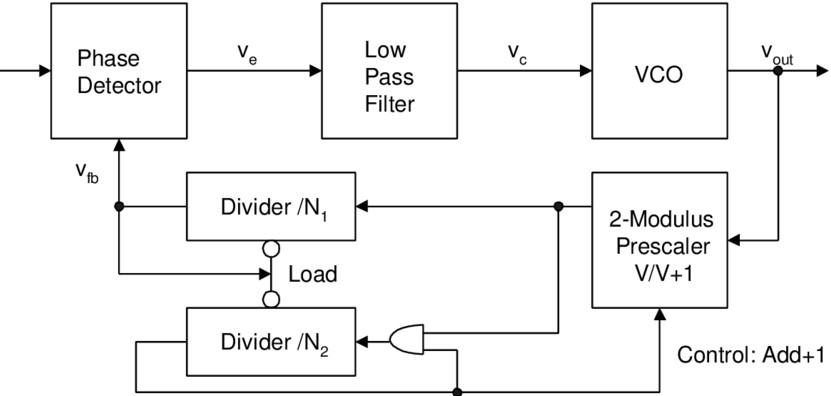Let’s extract programmable clock divider out of PLL circuitry as below. Dual modulus prescaler means the divide ratio it applies is either P or P+1 depending on the Modulus Control signal. Here P is fixed and normally small so it is easier to close timing on fast raw clock Fin. There are two other counters, N and A. Both are programmable but they run on the slower DMP divided clock. This circuit works on the condition that N is no less than A, aka N>=A.

The circuit works like this. At the beginning, DMP divides Fin by P+1 and both N and A counters start counting down. When A counter counts down to 0, A counter stops and this event triggers DMP to divide Fin by P. Since N>=A, N counter continues counting down and eventually reaches 0. This event will reload both N and A counter and also changes DMP divide ratio back to P+1. The whole process then repeats above cycle. Fout toggles once per this cycle.

As can be seen, in one Fout cycle, there are A*(P+1)+(N-A)*P=P*N+A Fin cycles elapsed. So the combined divide ratio is P*N+A.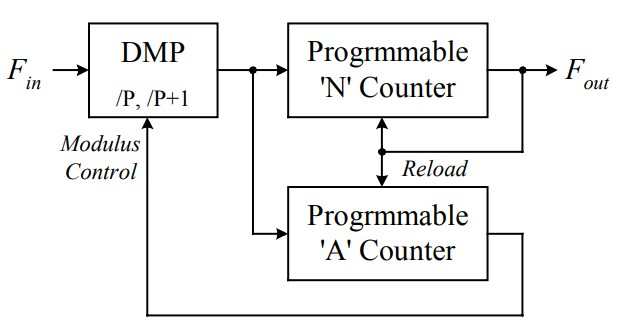Here is dual modulus divide-by-4/5 prescaler example.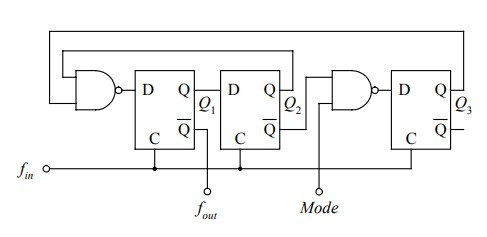Mode=0 is to divide by 4 and mode=0 is by 5. Here is the waveform.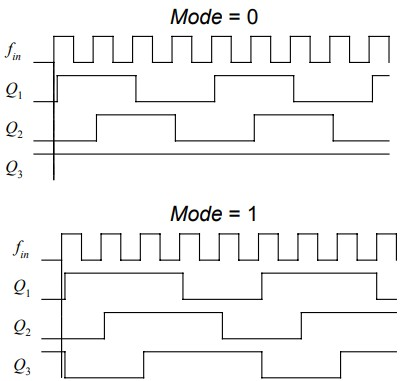So use this divide-by-4/5 prescaler, we can get 4*N+A divide ratio.

Here is another example of divide-by-2/3 prescaler. MC=0 is to divide by 3 and MC=1 is by 2 where only the 2nd FF is used and acts as a typical divide-by-2 circuit.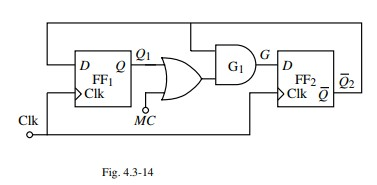If we are concerned prescaler generated clock is still too high, we can cascade DMPs as below.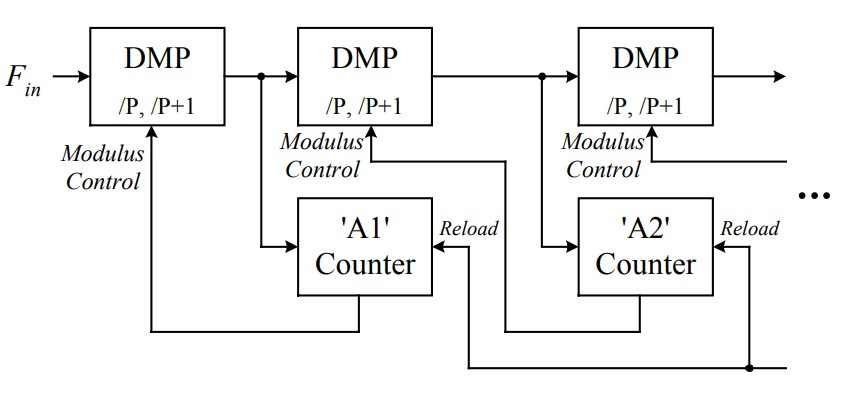In fact, cascading 2/3 cells can achieve below polynomial clock divider. I don’t know if it is of much use but it is fun!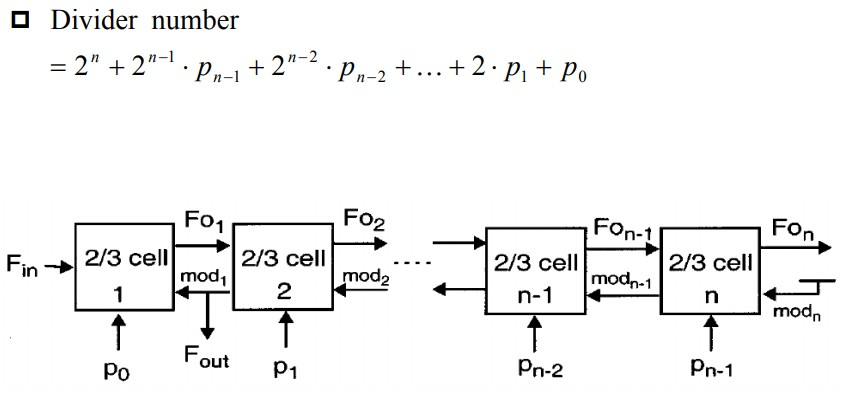Here is 2/3 cell used in above cascading circuit.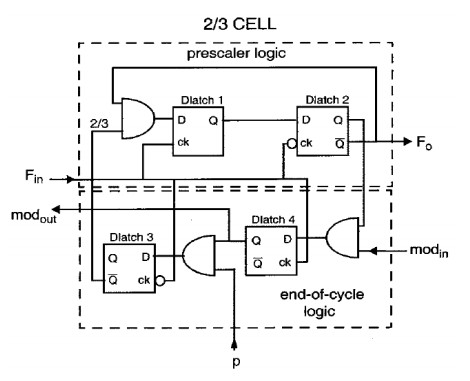Finally, DMP can also be used to achieve fractional clock divider in PLL. Below is the circuitry.  But i am not sure if it can work as a standalone fractional clock divider without those PLL blocks such as PFD/LPF/VCO.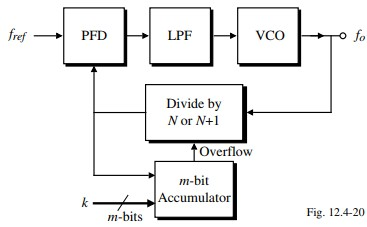Author brief is empty
Groups:

Tags: